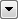You are here: Coordinates > ReprojectAngleCalculator

# ReprojectAngleCalculator

Converts a given angle from one coordinate system to another. The transformer uses the first coordinate of the passed-in feature and returns the angle of the specified line at the determined angle in the destination coordinate system. It is useful for converting data such as text rotations that are established in many systems in the destination coordinate system units.

To calculate the new angle, the transformer uses the:

• input length
• input angle
• input point
• destination (writer) coordinate system

and then calculates a second point in the source coordinate system by taking the first point and advancing length units in the angle heading. It then reprojects both points to determine the new angle.

## Usage Notes

This function assumes all coordinate systems are Cartesian.

## Editing Transformer Parameters

Using a set of menu options, transformer parameters can be assigned by referencing other elements in the workspace. More advanced functions, such as an advanced editor and an arithmetic editor, are also available in some transformers. To access a menu of these options, clickbeside the applicable parameter. For more information, see Transformer Parameter Menu Options.